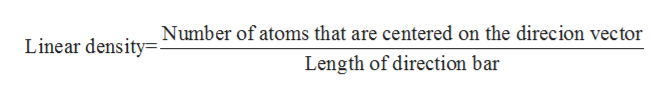Derive linear density expressions for BCC  and  directions in terms of the atomic radius R

Question

Derive linear density expressions for BCC  and  directions in terms of the atomic radius R

Step 1

In case of BCC type crystal structure, the atoms are arranged at the corners of the cube such that one atom being present at the center of the cube. Hence, the coordination number of the atoms in case of BCC is 8 and the packing efficiency is known to be 58%.

Step 2

The term linear density refers to the number of atoms present in per unit length where its center lies in the direction of the vector. The mathematical relation is shown below:help_outlineImage TranscriptioncloseLinear density Number of atoms that are centered on the direcion vector Length of direction bar fullscreen
Step 3

In the given problem the expression for the linear density in a BCC lattice is to be deri...

Want to see the full answer?

See Solution

Want to see this answer and more?

Our solutions are written by experts, many with advanced degrees, and available 24/7

See Solution
Tagged in

Physical Chemistry